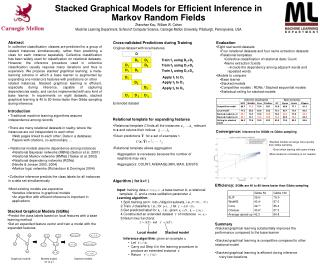DownloadDownload PresentationStacked Graphical Models for Efficient Inference in Markov Random Fields

# Stacked Graphical Models for Efficient Inference in Markov Random Fields

Télécharger la présentation## Stacked Graphical Models for Efficient Inference in Markov Random Fields

- - - - - - - - - - - - - - - - - - - - - - - - - - - E N D - - - - - - - - - - - - - - - - - - - - - - - - - - -
##### Presentation Transcript

1. x2,y’1 y’2 y’4 x3, y’4 y’3 x4, y’2 y’3 y’4 y’5 x1, y’1 y’2 x1, y’4 y’5 Stacked Graphical Models for Efficient Inference in Markov Random Fields Zhenzhen Kou, William W. Cohen Machine Learning Department, School of Computer Science, Carnegie Mellon University, Pittsburgh, Pennsylvania, USA Abstract In collective classification, classes are predicted for a group of related instances simultaneously, rather than predicting a class for each instance separately. Collective classification has been widely used for classification on relational datasets. However, the inference procedure used in collective classification usually requires many iterations and thus is expensive. We propose stacked graphical learning, a meta-learning scheme in which a base learner is augmented by expanding one instance's features with predictions on other related instances. Stacked graphical learning is efficient, especially during inference, capable of capturing dependencies easily, and can be implemented with any kind of base learner. In experiments on eight datasets, stacked graphical learning is 40 to 80 times faster than Gibbs sampling during inference. Cross-validated Predictions during Training • Evaluation • Eight real world datasets • Four relational datasets and four name extraction datasets • Relational templates • Collective classification of relational data: Count • Name extraction: Exists • Include the dependency among adjacent words and repeated words • Models to compare • Base learner • Stacked models • Competitive models : RDNs / Stacked sequential models • Statistical ceiling for stacked models Original dataset with local features D D2 D3 Train f1 using D2+D3 D1 D3 Train f2 using D1+D3 D1 D2 Train f3 using D1+D2 D1’ Apply f1 to D1 D2’ Apply f2 to D2 D3’ Apply f3 to D3 D’1 D’2 D’3 Extended dataset • Introduction • Traditional machine learning algorithms assume independence among records • There are many relational datasets in reality, where the instances are not independent to each other • Web pages linked to each other; Data in a database; Papers with citations, co-authorships; … • Relational models assume dependence among instances • Relational Bayesian networks (RBNs) (Getoor et al. 2001) • Relational Markov networks (RMNs) (Taskar et al. 2002) • Relational dependency networks (RDNs)(Neville & Jensen 2003, 2004) • Markov logic networks (Richardson & Domingos 2004) • Collective inference predicts the class labels for all instances in a data set simultaneously • Most existing models are expensive • Iterative inference in graphical models • An algorithm with efficient inference is important in applications • Relational template for expanding features • Relational template C finds all the instances relevant to x and returns their indices • Given predictions for a set of examples • Relational template allows aggregation • Aggregation is necessary because the number of neighbors may vary • Aggregators: COUNT, AVERAGE,MIN, MAX, EXISTS Convergence: inference for SGMs vs Gibbs sampling • Stacked models converge more quickly than Gibbs sampling • Even when starting with same initials • More iterations of stacking is not needed • Algorithm ( for k=1 ) • Input: training data , a base learner A, a relational template C, and a cross-validation parameter J. • Learning algorithm: • Split training set into J disjoint subsets, i.e., • Train J classifiers, i.e., for let • Get predicted label for , i.e., given • Constructed an extended dataset of instances • Return two functions:Inference algorithm: given an example • Let • Carry out Step 4 in the learning procedure to produce an extended instance • Return Efficiency: SGMs are 40 to 80 times faster than Gibbs sampling • Stacked Graphical Models (SGMs) • Predict the class labels based on local features with a base learning method • Get an expanded feature vector and train a model with the expanded features • Summary • Stacked graphical learning substantially improves the performance compared to the base learner • Stacked graphical learning is competitive compared to other relational model • Stacked graphical learning is efficient during inference • very few iterations Local model Stacked model x2 x3 x2, y’2 x3, y’3 x4 x4, y’4 x1 x1, y’1 x5 x1, y’5 Stacked models Graphical models Models based on (x,y’)